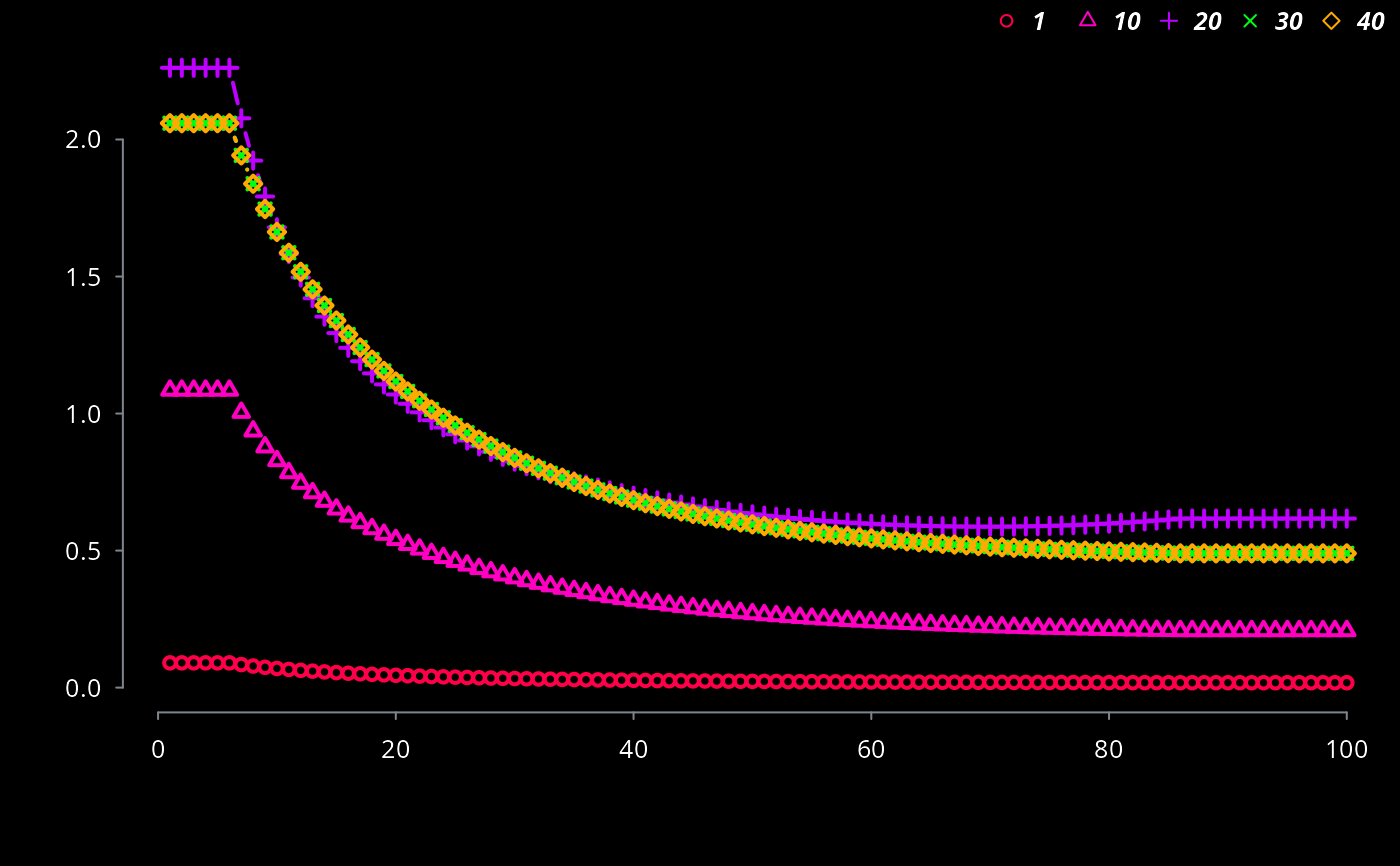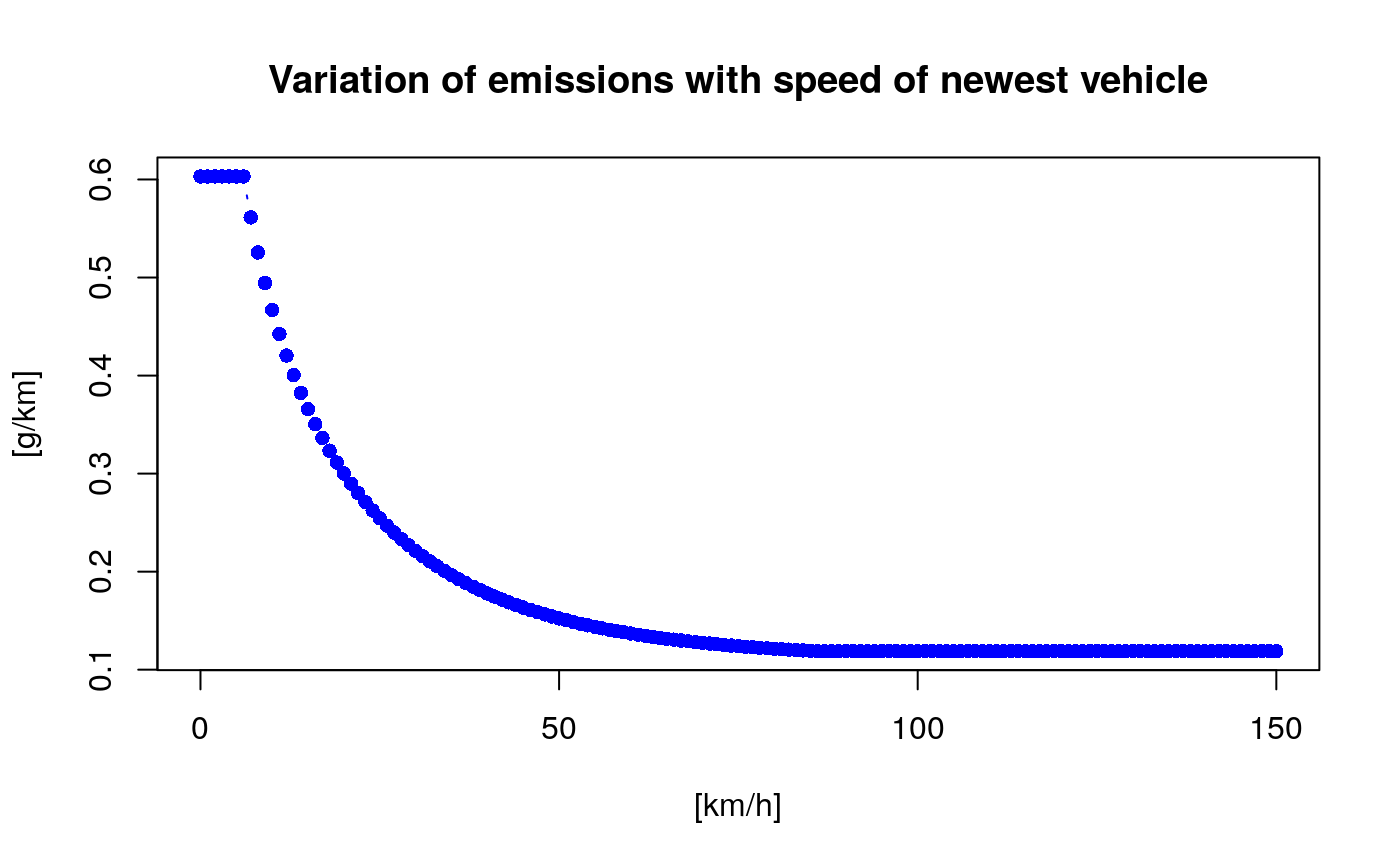ef_hdv_scaled creates a list of scaled functions of emission factors. A scaled emission factor which at a speed of the dricing cycle (SDC) gives a desired value. This function needs a dataframe with local emission factors with a columns with the name "Euro_HDV" indicating the Euro equivalence standard, assuming that there are available local emission factors for several consecutive years.

ef_hdv_scaled(df, dfcol, SDC = 34.12, v, t, g, eu, gr = 0, l = 0.5,
p)

df deprecated Column of the dataframe with the local emission factors eg df$dfcol Speed of the driving cycle Category vehicle: "Coach", "Trucks" or "Ubus" Sub-category of of vehicle: "3Axes", "Artic", "Midi", "RT, "Std" and "TT" Gross weight of each category: "<=18", ">18", "<=15", ">15 & <=18", "<=7.5", ">7.5 & <=12", ">12 & <=14", ">14 & <=20", ">20 & <=26", ">26 & <=28", ">28 & <=32", ">32", ">20 & <=28", ">28 & <=34", ">34 & <=40", ">40 & <=50" or ">50 & <=60" Euro emission standard: "PRE", "I", "II", "III", "IV" and "V" Gradient or slope of road: -0.06, -0.04, -0.02, 0.00, 0.02. 0.04 or 0.06 Load of the vehicle: 0.0, 0.5 or 1.0 Pollutant: "CO", "FC", "NOx" or "HC" Value A list of scaled emission factors g/km Note The length of the list should be equal to the name of the age categories of a specific type of vehicle Examples { # Do not run data(fe2015) co1 <- fe2015[fe2015$Pollutant=="CO",] lef <- ef_hdv_scaled(dfcol = co1$LT, v = "Trucks", t = "RT", g = "<=7.5", eu = co1$Euro_HDV, gr = 0, l = 0.5, p = "CO") length(lef) plot(x = 0:150, y = lef[](0:150), col = "red", type = "b", ylab = "[g/km]", pch = 16, xlab = "[km/h]", main = "Variation of emissions with speed of oldest vehicle") plot(x = 0:150, y = lef[](0:150), col = "blue", type = "b", ylab = "[g/km]", pch = 16, xlab = "[km/h]", main = "Variation of emissions with speed of newest vehicle") }